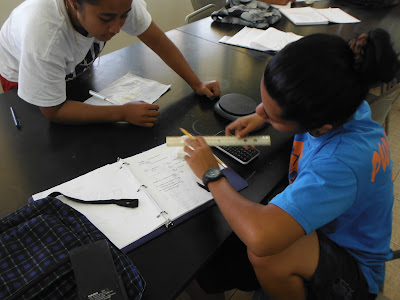## Posts

Showing posts from August, 2015

### Density of soap and speed of a ballThe fall term began with a laboratory that introduces measurement through the measurement of the density of soap.
Regina Moya measures her soap
I began the laboratory with the Freeman Dyson quote:

For a physicist mathematics is not just a tool by means of which phenomena can be calculated, it is the main source of concepts and principles by means of which new theories can be created... ...equations are quite miraculous in a certain way. ... the fact that nature talks mathematics, I find it miraculous. ... I spent my early days calculating very, very precisely how electrons ought to behave. Well, then somebody went into the laboratory and the electron knew the answer. The electron somehow knew it had to resonate at that frequency which I calculated. So that, to me, is something at the basic level we don't understand. Why is nature mathematical? But there's no doubt it's true. And, of course, that was the basis of Einstein's faith. I mean, Einstein talked that mathematic…